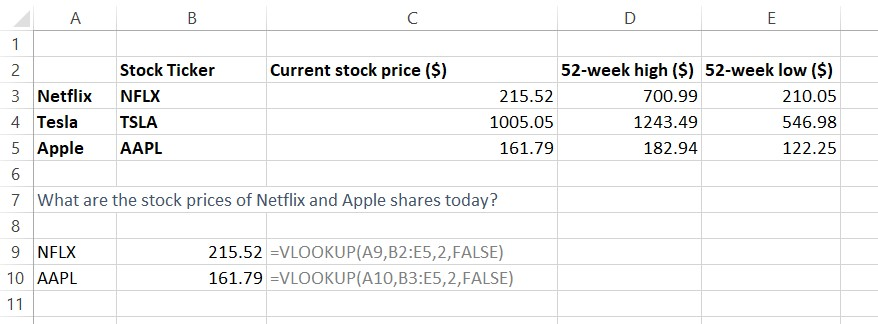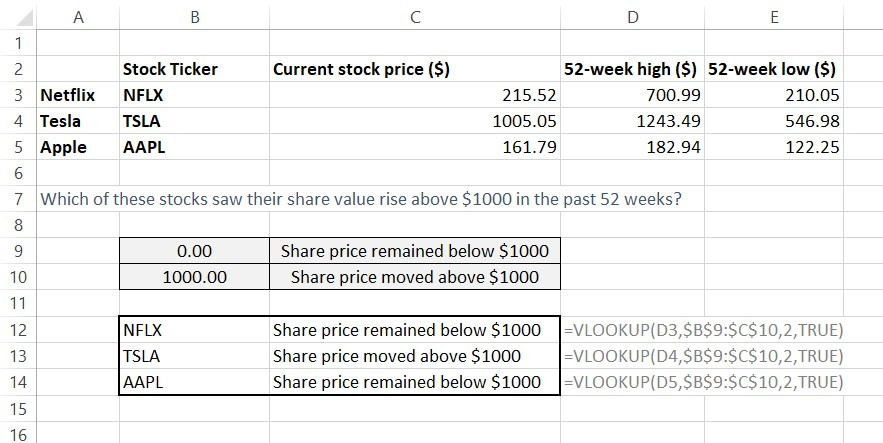## What is vlookup?

Vlookup is a function within excel which can be used for retrieving relevant information from a larger data set.

Frequently in finance modeling, there will be large data sets that you may work with. There might be moments when this data set on its own may not be useful, and you may need to pull out meaningful details.

For vlookup to work, the user must know the information in the first column, this is key for this formula to work correctly. The user must also know which column excel needs to search the information which is to be retrieved. Excel then returns the information in the cell which overlaps the row and column which is inserted in the formula.

Vlookup is not case sensitive, it will not differentiate between “Sales” and “sales”. Vlookup also has two modes of searching, first being the exact match, where excel will need the exact value in column one to conduct the search, this is useful for when you may have the exact unique value in column one. Vlookup can also work in approximate mode, which is necessary when working in a number range.

## SYNTAX

Vlookup function appears on excel as:

=Vlookup(lookup_value, table_array, Col_index_number, [range_lookup], FALSE)Vlookup function appears on excel as: =Vlookup(lookup_value, table_array, Col_index_number, [range_lookup], FALSE)

The simplified explanation of each of those is as follows:

• lookup_value: what you want to look up, excel will search this in the first column of the selected table.
• table_array: the table in which you wish to look up
• col_index_number: the number of the column to be searched, within the range that you selected.
• range_lookup: there are two options which can be selected in this section, FALSE or TRUE. This will tell excel if it needs to look for the exact figure (FALSE) or an approximate figure (TRUE). False can also be written as 0 and true can also be written as 1.

Vlookup will return the value in the overlapping column and row cell, as specified in the formula. Vlookup stands for “vertical lookup”, excel will search for information in a vertical column once the lookup value is inserted.

## Key Learning Points

• Vlookup is an excel function that allows the user to pull out relevant information from a larger data set.
• For the formula to work, the user must know the information in the first column, and the number of the column excel needs to search for the information.
• Vlookup is not case sensitive.
• You can search exact matches or approximate matches.

## How to use vlookup

A simple way to think about how vlookup works is to think of it like looking up information in a telephone book. In a situation where you may already know the name of an individual, you can use the vlookup function to find their phone number.

Within finance this function can come in useful when looking up information in a large table. Using this function can not only make financial models more dynamic, but can also save a lot of time when searching for information within a large table. Data extraction

The two modes of the function, the true and approximate match, can also be creatively used within models, each producing slightly different results, as shown below.

## Example of an exact match

In the example below, we have used the stock ticker as our search key, to look up the relevant share price information.## Example of an approximate match

When using vlookup in an approximate mode, the formula is used slightly differently.

In the example below, excel is being asked to search a number in a range, and return information from a table which contains relevant criteria. The user will need to create this separate table with the unique criteria.